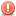CodeGuru Home VC++ / MFC / C++ .NET / C# Visual Basic VB Forums Developer.com

# Thread: working with multiple directories with VBA in excel

1.Junior MemberJoin Date
Jun 2010
Posts
4

##working with multiple directories with VBA in excel

I have run into a small problem with a current project I am working on. Bassically what the project will do is open multiple files in multiple directories and reformat some files and combine data between the files to create some reports.

The problem I am having is that when i go to cycle through the the second set of files while cycling through the first set my code seems to "forget" or lose its place within the first directory it is cycling through.

Here is all the code from my project.. the problem occurs during "filename = dir()"

Code:
```Option Explicit

'32-bit API declarations
Declare Function SHGetPathFromIDList Lib "shell32.dll" _
Alias "SHGetPathFromIDListA" (ByVal pidl As Long, ByVal _
pszpath As String) As Long

Declare Function SHBrowseForFolder Lib "shell32.dll" _
Alias "SHBrowseForFolderA" (lpBrowseInfo As BrowseInfo) _
As Long

Public Type BrowseInfo
hOwner As Long
pIDLRoot As Long
pszDisplayName As String
lpszTitle As String
ulFlags As Long
lpfn As Long
lParam As Long
iImage As Long
End Type

Function GetDirectory(Optional msg) As String
On Error Resume Next
Dim bInfo As BrowseInfo
Dim path As String
Dim r As Long, x As Long, pos As Integer

'Root folder = Desktop
bInfo.pIDLRoot = 0&

'Title in the dialog
If IsMissing(msg) Then
bInfo.lpszTitle = "Please select the folder of the excel files to copy."
Else
bInfo.lpszTitle = msg
End If

'Type of directory to return
bInfo.ulFlags = &H1

'Display the dialog
x = SHBrowseForFolder(bInfo)

'Parse the result
path = Space\$(512)
r = SHGetPathFromIDList(ByVal x, ByVal path)
If r Then
pos = InStr(path, Chr\$(0))
GetDirectory = Left(path, pos - 1)
Else
GetDirectory = ""
End If
End Function

Sub CombineFiles()

Dim LastCell        As Range
Dim Wkb, Wkb2            As Workbook
Dim WS, WS2              As Worksheet
Dim ThisWB, d, m, fd, tex, path, pathmd, FileName, FileName2  As String
Dim md As Integer

If UNameWindows() <> "speelb" Then
MsgBox "Only run this while Brian S. is logged in"
Exit Sub
End If
Sheet1.Range("A42").Copy
Sheet1.Range("A41").PasteSpecial Paste:=xlPasteValuesAndNumberFormats, Operation:=xlNone, SkipBlanks:=False, Transpose:=False
ThisWB = ThisWorkbook.Name
Application.EnableEvents = False
Application.ScreenUpdating = False
path = GetDirectory
pathmd = GetDirectory
FileName2 = Dir(pathmd & "\*.xls", vbNormal)
FileName = Dir(path & "\*.xls", vbNormal)

Do Until FileName = ""
If FileName <> ThisWB Then
Set Wkb = Workbooks.Open(FileName:=path & "\" & FileName)
For Each WS In Wkb.Worksheets
Set LastCell = WS.Cells.SpecialCells(xlCellTypeLastCell)

'cover page procedure
If WS.Name = "Cover Page" Then

md = Sheet1.Range("a45").Value
If Sheet1.Range("A44") = 1 Then
m = "January"
ElseIf Sheet1.Range("A44") = 2 Then
m = "February"
ElseIf Sheet1.Range("A44") = 3 Then
m = "March"
ElseIf Sheet1.Range("A44") = 4 Then
m = "April"
ElseIf Sheet1.Range("A44") = 5 Then
m = "May"
ElseIf Sheet1.Range("A44") = 6 Then
m = "June"
ElseIf Sheet1.Range("A44") = 7 Then
m = "July"
ElseIf Sheet1.Range("A44") = 8 Then
m = "August"
ElseIf Sheet1.Range("A44") = 9 Then
m = "September"
ElseIf Sheet1.Range("A44") = 10 Then
m = "October"
ElseIf Sheet1.Range("A44") = 11 Then
m = "November"
ElseIf Sheet1.Range("A44") = 12 Then
m = "December"
End If

If Sheet1.Range("A43") = 1 Then
d = "Sunday"
ElseIf Sheet1.Range("A43") = 2 Then
d = "Monday"
ElseIf Sheet1.Range("A43") = 3 Then
d = "Tuesday"
ElseIf Sheet1.Range("A43") = 4 Then
d = "Wednesday"
ElseIf Sheet1.Range("A43") = 5 Then
d = "Thursday"
ElseIf Sheet1.Range("A43") = 6 Then
d = "Friday"
ElseIf Sheet1.Range("A43") = 7 Then
d = "Saturday"
End If

fd = d & ", " & m & " " & md

tex = Sheet1.Range("A38") & fd & Sheet1.Range("A39")

Sheet1.Range("a11") = tex

With Sheet1.Range("a11").Characters(Start:=87, Length:=Len(fd)).Font
.Name = "Arial"
.FontStyle = "Bold Italic"
.Size = 10
.Strikethrough = False
.Superscript = False
.Subscript = False
.OutlineFont = False
.Underline = xlUnderlineStyleNone
.Color = -16776961
.ThemeFont = xlThemeFontNone
End With
ThisWorkbook.Sheets("Sheet1").Range("A1:B31").Copy Destination:=WS.Range("A18:B48")
WS.Columns("A:B").EntireColumn.AutoFit
WS.Rows("18:48").EntireRow.AutoFit
WS.Rows(28).RowHeight = 32
WS.PageSetup.Zoom = 60
WS.Activate
ActiveWindow.Zoom = 80
End If
Dim nam As String
Do Until FileName2 = ""

nam = Right(FileName2, Len(FileName2) - 1)
If nam = Wkb.Name Then
Set Wkb2 = Workbooks.Open(FileName:=pathmd & "\" & FileName2)
For Each WS2 In Wkb2.Worksheets

Else
WS2.Copy After:=Wkb.Sheets(Wkb.Sheets.Count)
End If
Next WS2
Wkb2.Close False
End If
FileName2 = Dir()
Loop
'MD page procedure
If WS.Name = "MD" Then
' need to change format, delete columns, delete rows, add in formulas, cross check with chksmp and pull in names
End If
'     MsgBox FileName

'                Else
'                    WS.Copy After:=ThisWorkbook.Sheets(ThisWorkbook.Sheets.Count)
'                End If
Next WS
Wkb.Save
Wkb.Close False
End If
MsgBox FileName
FileName = Dir()
Loop
Application.EnableEvents = True
Application.ScreenUpdating = True

Set Wkb = Nothing
Set LastCell = Nothing
End Sub

Function UNameWindows() As String
End Function

Public Function TrailingSlash(strFolder As String) As String
If Len(strFolder) > 0 Then
If Right(strFolder, 1) = "\" Then
TrailingSlash = strFolder
Else
TrailingSlash = strFolder & "\"
End If
End If
End Function```
Last edited by bspeel; July 1st, 2010 at 11:56 AM.Reply With Quote

dir, directories, excel, multiple files, vba####Posting Permissions

• You may not post new threads
• You may not post replies
• You may not post attachments
• You may not edit your posts
•